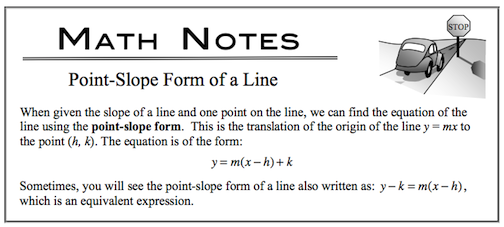### Home > CALC > Chapter 5 > Lesson 5.4.1 > Problem5-145

5-145.

Find the equation of the line tangent to $y =\operatorname{cot}(2x)$ at $x = \frac { \pi } { 3 }$.

Use point-slope form of the line.Find the slope using the derivative at the same point.